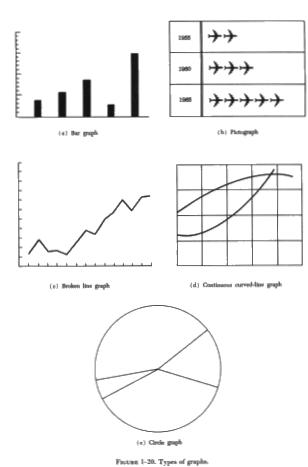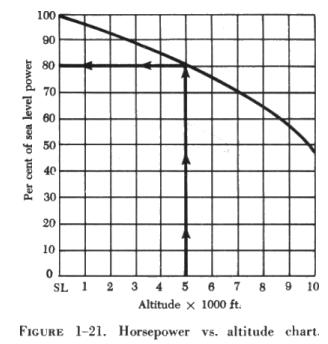GRAPHSANDCHARTS
 GRAPHS AND CHARTS Graphs and charts are pictorial presentations of data, equations, and formulas. Through their use the relationship between two or more quantities may be more clearly understood. Also, a person can see certain conditions or relationships at a glance, while it would require considerable time to obtain the same information from a written description. Graphs may be used in a number of ways, such as representing a single equation or formula, or they may be used to solve two equations for a common value. Graphs and charts take many forms. A few of the more common forms are called bar graphs, pictographs, broken line graphs, continuous curved line graphs, and circle graphs. An example of each is shown in figure 1-20. The most useful of these graphs in technical work is the continuous curved line graph.   Interpreting/Reading Interpreting or Reading Graphs and ChartsIt is more important, from the mechanic's viewpoint, to be able to read a graph properly than it is to draw one. The relationship between the horsepower of a certain engine at sea level and at any altitude up to 10,000 feet can be determined by use of the chart in figure 1-21. To use this type of chart, simply find the point on the horizontal axis that represents the desired altitude; move upward along this line to the point where it intersects the curved line; then move to the left, reading the percent of sea level horsepower available on the vertical axis. EXAMPLE What percent of the sea level horsepower is available at an altitude of 5,000 feet? FIRST: Locate the point on the horizontal axis that represents 5,000 feet. Move upward to the point where the line intersects the curved line.

NEXT: Move to the left, reading the percent of sea level horsepower available at 5,000 feet. The available horsepower is 80%.

Nomograms

It is often necessary to make calculations using the same formula, but using different sets of values for the variables. It is possible to obtain a solution by use of a slide rule or by preparing a table giving the solution of the formula resulting from successive changes of each variable. However, in the case of formulas involving several mathematical operations, the labor entailed would usually be very great.

It is possible to avoid all this labor by using a diagram representing the formula, in which each variable is represented by one or more graduated lines. From this diagram, the solution of the formula for any given variable may be read by means of an index line. A diagram of this type is known as a nomogram.

Much of the information needed to solve aeronautical problems will be presented in nomogram form. Instruction manuals for the various aircraft contain numerous nomograms, many of which appear quite complex.

Many of the presentations will possess several curves on the same coordinate axis, each curve drawn for different constants in the equation. In the latter case, it is essential to select the proper curve for the desired conditions.

Again, as with the simpler graphs, it is more important for the mechanic to be able to read nomograms than it is to draw them.

The following example is taken from the maintenance manual for the Allison 501-D13 turboprop engine. A nomogram (figure 1-22) is used to determine the power requirements when the engine is operating at minimum torque. The OAT (outside air temperature), station barometric pressure, and engine rpm are three factors that must be known to use this particular nomogram.EXAMPLE

Determine the calculated horsepower of a certain engine, using the nomogram in figure 1-22. Assume that the OAT is 10° C, the barometric pressure is 28.5 in Hg, and the engine is operating at 10,000 rpm.

FIRST: Locate the reference points on the OAT scale and on the barometric pressure scale that correspond to the given temperature and pressure readings. These are identified as 1 and 2 respectively, on the chart. With the aid of a straightedge, connect these two points and establish point 3 on the pivot line.

NEXT: Locate the engine speed, identified as 4 on the engine speed rpm scale. Using a straightedge, connect points 3 and 4 and establish point 5 on the calculated horsepower scale. The calculated horsepower is read at point 5. The calculated horsepower is 98%.